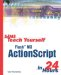# Loops

 I l @ ve RuBoard

### Loops

The syntax for creating loops in ActionScript is a little more complex than the simple if statement. Fortunately, it is almost identical to how loops are created in languages such as C, C++, and Java.

#### The for Loop

The primary type of loop is the for loop. It looks like this:

` for(var i=0;i<10;i++) {     trace(i); } `

If you run this code in a frame script, you get the numbers 0 through 9 placed in the Output window. The loop counts from 0 to 9, changing the local variable i along the way.

As you can see, a for statement has three parts . Each part is separated by a semicolon.

The first part is a variable declaration. In this case, the local variable i is created and set to 0. This first part of the for statement is executed once, before the loop starts.

The second part of the for statement is a condition. In this case, it tests to see whether i is less than 10. The for loop continues to run as long as this condition is true. When the loop starts, i is equal to 0, which is certainly less than 10, so the loop begins.

The third part of the for statement is an operation to be performed every time the loop loops. In this case, i is incremented by 1. This operation is performed after each iteration of the loop because the ++ operator is placed after the i . If it were placed before the i , such as ++i , the operation would take place before the commands inside the loop are executed.

Inside the brackets are the commands to be executed each time through the loop. Let's play computer and see how the example works:

1. The local variable i is created and set to 0.

2. A check is made to make sure that i is less than 10. Because it is, the loop is allowed to continue.

3. A note is made that the variable i should be incremented by 1 when each iteration of the loop is complete. For now, i remains at 0.

4. The trace command then sends the contents of i to the Output window, in this case 0.

5. The iteration of the loop ends, and i is increased by 1.

6. The loop starts again, and the check is made to see whether i is less than 10. It is, because i is now 1, and the loop is allowed to continue.

7. The trace command sends the contents of i to the Output window, in this case 1.

This continues, with i increasing by 1 each time, until the 10th time through the loop. Then the following happens:

1. The iteration of the loop ends, and i is increased by 1. Its value is now 10.

2. The loop starts again, and the check is made to see whether i is less than 10. It is not because i is equal to 10. The loop ends.

3. The next line after the closing bracket at the end of the loop executes, and the loop is over.

#### Other Kinds of Loops

The for loop is the most common kind of loop. However, two other kinds of loops are the while loop and the do loop.

The while loop looks like this:

` while (a != 7) {     // more code here } `

As you can see, this is a much simpler loop than a for loop. It actually looks just like an if statement, except that the code in the brackets will continue to run over and over again until the condition is met. This being the case, it is easy to create undesirable infinite loops. You would have to make sure that the code inside the loop alters a in some way so that it eventually achieves the value of 7 and the loop ends.

The sibling to the while loop is the do loop. Here is what it looks like:

` do {     // more code here }  while (a != 7); `

The while and do loops are actually the same thing, except that the condition is checked in different places. In the while loop, the condition is checked before each iteration of the loop, whereas in the do loop, it is checked after each iteration of the loop. The difference is that the do loop always runs at least once.

#### Breaking Out of Loops

All three kinds of loops can use two optional commands to change the flow of the loop. The first command, break , stops the loop and jumps right to the instruction following the loop.

The other command, continue , terminates the current pass through the loop but starts the next pass through the loop right away.

For instance, if instructions A, B, and C are inside the loop, and instruction B performs a continue command if a certain condition is met, instruction C will be skipped , and the loop will start again at A. If it was a break command instead, C would be skipped and the loop would end.

It is difficult to show valid examples of break and continue without a complex example, so we will save that for later in the book when we need it.I l @ ve RuBoardSams Teach Yourself Flash MX ActionScript in 24 Hours
ISBN: 0672323850
EAN: 2147483647
Year: 2002
Pages: 272

flylib.com © 2008-2017.
If you may any questions please contact us: flylib@qtcs.net